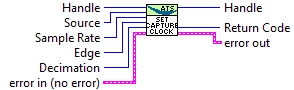# AlazarSetCaptureClock#

## Function Syntax#

RETURN_CODE AlazarSetCaptureClock(HANDLE handle, U32 source, U32 sampleRateIdOrValue, U32 edgeId, U32 decimation)#

Configure the sample clock source, edge and decimation.

• If a board is configured to use a SLOW_EXTERNAL_CLOCK clock source, the maximum decimation value is 1.

• If an ATS9350 is configured to use an EXTERNAL_CLOCK_10MHZ_REF clock source, the decimation value must be 1, 2, 4 or any multiple of 5. Note that the sample rate identifier value must be 500000000, and the sample rate will be 500 MHz divided by the decimation value.

• If an ATS9360 / ATS9371 / ATS9373 is configured to use an EXTERNAL_CLOCK_10MHZ_REF clock source, the maximum decimation value is 1.

• If an ATS9850 is configured to use an EXTERNAL_CLOCK_10MHZ_REF clock source, the decimation value must be 1, 2, 4 or any multiple of 10. Note that the sample rate identifier value must be 500000000, and the sample rate will be 500 MHz divided by the decimation value.

• If an ATS9870 is configured to use an EXTERNAL_CLOCK_10MHZ_REF clock source, the decimation value must be 1, 2, 4 or any multiple of 10. Note that the sample rate identifier value must be 1000000000, and the sample rate will be 1 GHz divided by the decimation value.

Parameters
• handle[in] Board handle

• source[in] Clock source identifiers. Must be a member of ALAZAR_CLOCK_SOURCES. See board-specific information for a list of valid values for each board. For external clock types, the identifier to choose may depend on the clock’s frequency. See board-specific information for a list of frequency ranges for all clock types.

• sampleRateIdOrValue[in] If the clock source chosen is INTERNAL_CLOCK, this value is a member of ALAZAR_SAMPLE_RATES that defines the internal sample rate to choose. Valid values for each board vary. If the clock source chosen is EXTERNAL_CLOCK_10MHZ_REF, pass the value of the sample clock to generate from the reference in hertz. The values that can be generated depend on the board model. Otherwise, the clock source is external, pass SAMPLE_RATE_USER_DEF to this parameter.

• edgeId[in] The external clock edge on which to latch sample rate. Must be a member of ALAZAR_CLOCK_EDGES.

• decimation[in] Decimation value. May be an integer between 0 and 100000 with the following exceptions. Note that a decimation value of 0 means disable decimation.

Returns

ApiSuccess upon success, or an error code. See RETURN_CODE for more detailed information.

## LabVIEW Block Diagram#